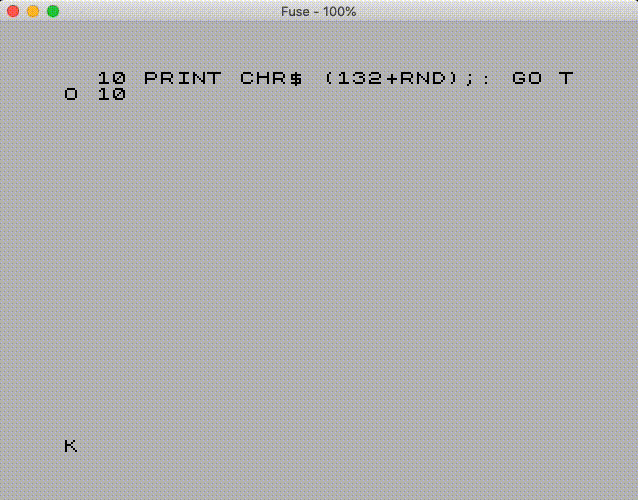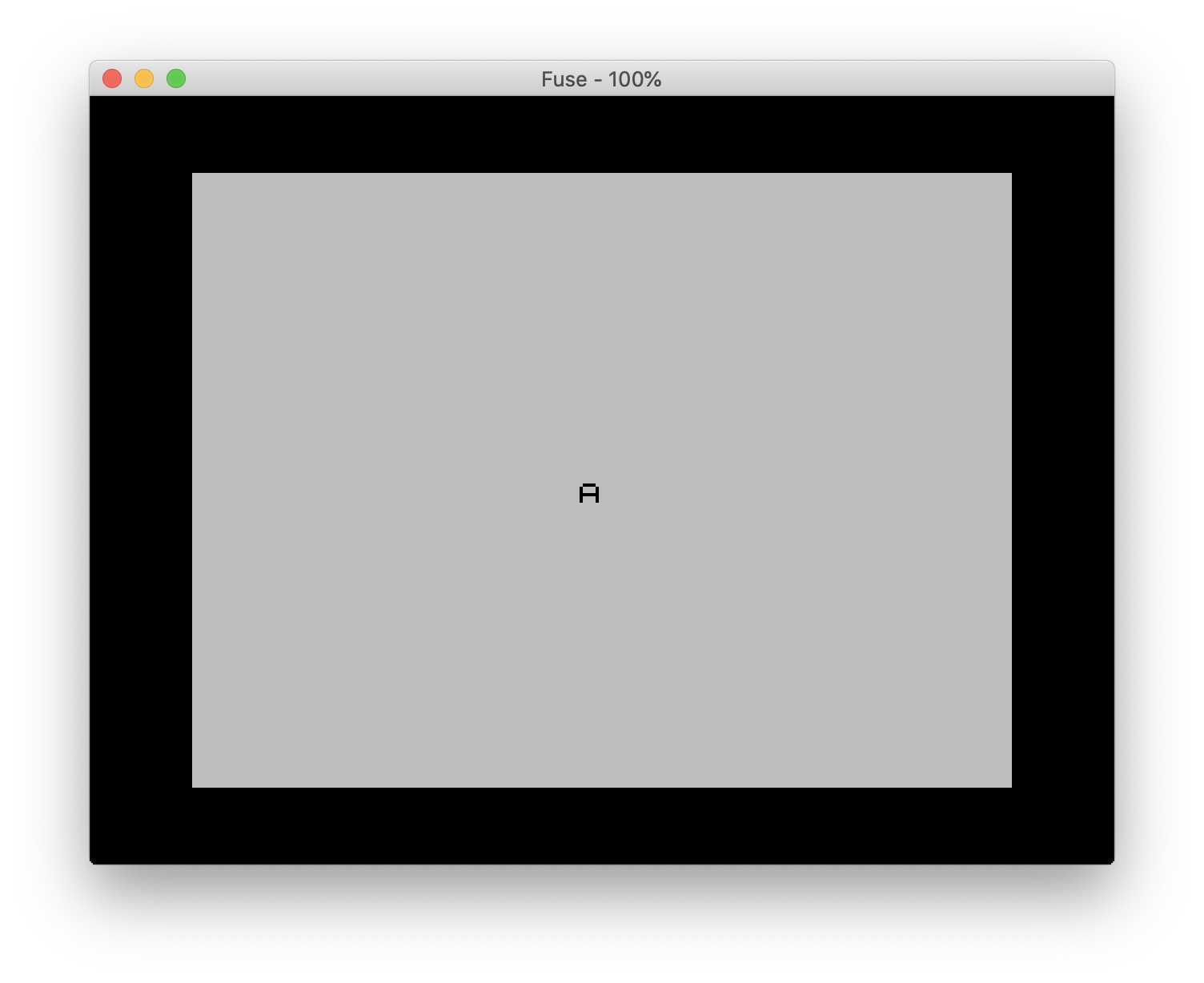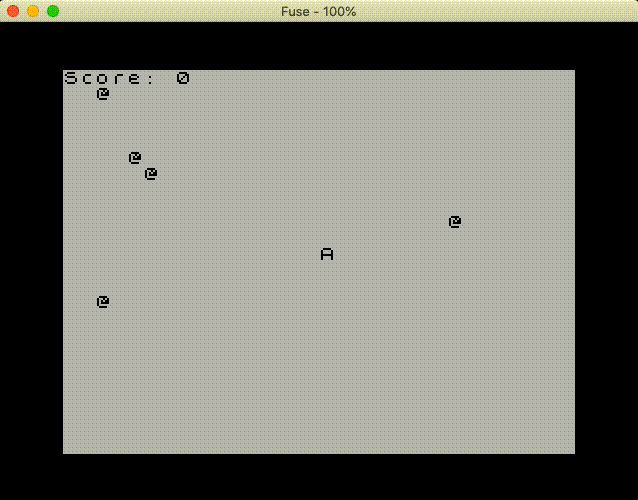Lars Diget

# Making a ZX Spectrum Game - Part 2 - The Initial Prototype in Sinclair Basic

Nov 17, 2020 10:45 · 1281 words · 7 minute read

Now it is time to write some actual code. As I described in a previous post, I want to create an initial prototype directly in Sinclair BASIC, which will allow me to test out the gameplay of the Awakeman game on a ZX Spectrum.

It has been a while since I coded in BASIC. My first job as a developer was to create small programs in Visual Basic for Applications (VBA), so building this prototype will also act as a refresher to BASIC.

Since I do not want to write the code itself on the ZX Spectrum, I will use a small tool called zmakebas so that I can move the BASIC code that I write on my Mac to a TAP file that I can use on the Fuse emulator or the real hardware.

Disclaimer: If you are following these tutorials, I expect you to understand programming in general. I will not explain the code itself in detail; it should be self-explanatory.

## Hello World

To see if everything works as expected, I made a file called test.bas, and add the following line of code:

``````10 PRINT CHR\$(132+RND);:GO TO 10
``````

Use the zmakebas tool to make the TAP file:

``````zmakebas test.bas
``````

Load the out.tab file into the emulator and press R and then Enter to run the code. If you see a maze very slowly printed on the screen, the test was successful.The 10 PRINT Maze Example Running in the Fuse emulator

If you want to learn more about the “10 PRINT” BASIC example used here, there is a book that goes into great detail about its origin and cultural context.

## Let’s Make the Prototype

To recap the game’s premise, you play as Awakeman, where the objective is to collect limes. For each lime you pick up, you earn a point.

For this initial prototype, I will primarily investigate how to move the character around on the screen. On the ZX Spectrum, it is standard to use the Q, A, O, and P buttons for up, down, left, and right.

I will need a couple of variables; an x – and y position of the character on screen and an indication of the direction the character is moving (vx and vy). I use PRINT AT to display where the character, “A”, is on the screen.

``````100 REM Init Game
101 LET x = 16
102 LET y = 11
110 BORDER 0

200 REM Main Game Loop
201 PRINT AT y,x;"A"

210 LET vx = 0
211 LET vy = 0

300 GOTO 200
``````

If you run the above code, you should see the “A” in the screen center, with a black border around.First prototype running in the Fuse emulator

We now got the basic code structure of our game, an initialization routine, and a game loop, where we update the screen and set the character’s direction.

## Adding Keyboard Support

Let’s look at how to support keyboard input. For that, Sinclair BASIC exposes the INKEY\$ function.

Based on the key-press, I set the direction of the character (vy or vx). If no movement is detected, we jump backward in the game loop (without updating the screen). If we detect a movement, we remove the character at the current position, update the x – and y position, and jump to the beginning of the game loop to redraw the screen.

``````212 LET k\$ = INKEY\$
213 IF k\$ = "q" THEN LET vy=-1
214 IF k\$ = "a" THEN LET vy=1
215 IF k\$ = "o" THEN LET vx=-1
216 IF k\$ = "p" THEN LET vx=1

250 IF vx = 0 AND vy = 0 THEN GOTO 210

260 PRINT AT y,x;" "
270 LET x = x+vx
280 LET y = y+vy

300 GOTO 200
``````

If you would run the program, you should now be able to move the character “A” using the Q, A, O, and P keys. The character will act weird, or the game will crash if it passes outside the screen’s bounds, so let’s fix this.

``````270 LET x = x+vx
271 IF x < 0 THEN LET x = 0
272 IF x > 31 THEN LET x = 31
280 LET y = y+vy
281 IF y < 1 THEN LET y = 1
282 IF y > 21 THEN LET y = 21
``````

The code will check if the character tries to pass the screen bounds and re-adjust the x – and y position accordingly. The ZX Spectrum screen is 32 columns (0..31) times 24 rows (0..23). However, with Sinclair BASIC, you cannot normally PRINT on the bottom two rows; hence the bottom y bound is set at 21. Also, I have set the top y bound to 1 to have a score indicator on the first row.

## Score Counter and Limes

Let’s implement the score indicator:

``````103 LET s = 0

202 PRINT AT 0,0;"Score: ";s
``````

I need a global variable s to contain the score, and I need to update the score counter within the game loop’s screen update routine. To test it out, let’s add some limes to the screen.

``````150 REM Generate Lemons
151 FOR l=1 to 5
152 PRINT AT INT(RND*20)+1,INT(RND*31);"@"
153 NEXT l

290 IF SCREEN\$(y,x) = "@" THEN LET s = s+1:PRINT AT INT(RND*20)+1,INT(RND*31);"@"
``````

Five limes, indicated with the @-symbol, are randomly scattered across the screen as part of the init routine. When moving the character, I check if there is a lime on the new position, if so, I add 1 to the score counter and randomly places a new lime on the screen.

When you run the code, you are now able to move the character in all directions and earn a point when the character picks up a lime:Running the complete prototype in the Fuse emulator

## Evaluating the Code

Here is the code in its entirety:

``````100 REM Init Game
101 LET x = 16
102 LET y = 11
103 LET s = 0
110 BORDER 0

150 REM Generate Lemons
151 FOR l=1 to 5
152 PRINT AT INT(RND*20)+1,INT(RND*31);"@"
153 NEXT l

200 REM Main Game Loop
201 PRINT AT y,x;"A"
202 PRINT AT 0,0;"Score: ";s

210 LET vx = 0
211 LET vy = 0
212 LET k\$ = INKEY\$
213 IF k\$ = "q" THEN LET vy=-1
214 IF k\$ = "a" THEN LET vy=1
215 IF k\$ = "o" THEN LET vx=-1
216 IF k\$ = "p" THEN LET vx=1

250 IF vx = 0 AND vy = 0 THEN GOTO 210

260 PRINT AT y,x;" "

270 LET x = x+vx
271 IF x < 0 THEN LET x = 0 272 IF x > 31 THEN LET x = 31

280 LET y = y+vy
281 IF y < 1 THEN LET y = 1 282 IF y > 21 THEN LET y = 21

290 IF SCREEN\$(y,x) = "@" THEN LET s = s+1:PRINT AT INT(RND*20)+1,INT(RND*31);"@"

300 GOTO 200
``````

I am impressed; it was possible to implement the basic game logic in 29 lines of code. The game is very crude, even for a ZX Spectrum game, but a lot is also missing. The game would benefit from having actual graphics and possibly also animations. Preferably, it should be possible to move the character diagonally. Currently, you can only move the character in a straight line due to the limitations of INKEY\$ (only detecting the last single key-press). Lastly, it should also be possible to use the entire screen within the border as the game’s play area.

Next, I will investigate how to add some graphics to the prototype before I move the project to – and compile it with ZX BASIC.